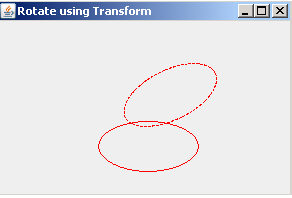Tutorials

# Show Rotation property of class AffineTransform

To show rotation, we have used Ellipse2D class to draw the shape oval. The method getTranslateInstance() returns the translation transformation.

To show rotation, we have used Ellipse2D class to draw the shape oval. The method getTranslateInstance() returns the translation transformation.

# Show Rotation property of class AffineTransform

This section shows you the rotation property.

To show rotation, we have used Ellipse2D class to draw the shape oval. The method getTranslateInstance() returns the translation transformation. Following code move the origin to 50,50:

 AffineTransform affineTransform = AffineTransform.getTranslateInstance(50, 50); g2d.transform(affineTransform);

The method draw(shape) draws the specified shape in the original location defined.

Following code transform the Graphics2D:

 g2d.transform(AffineTransform.getRotateInstance(-Math.PI / 7));

The stylistic representation of the outline for the specified shape has been given by the interface Stroke. To outline the graphics primitives, which are rendered with an object of Graphics2D, we have used the BasicStroke class, which defines a set of rendering attributes. The BasicStroke.CAP_BUTT gives the dash segments. The BasicStroke.JOIN_BEVEL joins the path segments by connecting the outer corners of their wide outlines with a straight segment.

Following code draws the specified shape in dashed format:

 Stroke stroke = new BasicStroke(1, BasicStroke.CAP_BUTT, BasicStroke.JOIN_BEVEL, 0, new float[] { 3, 1 }, 0); g2d.setStroke(stroke); g2d.draw(shape);

The method setStroke() sets the stroke settings for the Graphics2D context, when you draw the shape.

Here is the code of RotateWithTransform.java

 ``` import java.awt.*; import javax.swing.*; import java.awt.geom.*; public class RotationWithTransform extends JComponent {   Shape shape;   public RotationWithTransform() {   shape = create();   }   protected Shape create() {   float fl = 100 / 2.00f;   return new Ellipse2D.Float(fl, fl, 2 * fl, fl);   }    public void paint(Graphics g) {   Graphics2D g2d = (Graphics2D) g;   AffineTransform affineTransform = AffineTransform.   getTranslateInstance(50, 50);   g2d.transform(affineTransform);   g2d.setPaint(Color.red);   g2d.draw(shape);   g2d.transform(AffineTransform.getRotateInstance(-Math.PI / 7));   Stroke stroke = new BasicStroke(1, BasicStroke.CAP_BUTT,   BasicStroke.JOIN_BEVEL, 0, new float[] { 3, 1 }, 0);   g2d.setStroke(stroke);   g2d.draw(shape);   }   public static void main(String[] a) {   JFrame frame = new JFrame("Rotate using Transform");   frame.getContentPane().add(new RotationWithTransform());   frame.setSize(300, 200);   frame.show();   } }```

Output will be displayed as: